## 68. Datatype ConstructorsUp: Derived Datatypes Next: Subarray Datatype Constructor Previous: Type Constructors with Explicit Addresses

Contiguous The simplest datatype constructor is MPI_TYPE_CONTIGUOUS which allows replication of a datatype into contiguous locations.

 MPI_TYPE_CONTIGUOUS(count, oldtype, newtype) IN count replication count (nonnegative integer) IN oldtype old datatype (handle) OUT newtype new datatype (handle)

int MPI_Type_contiguous(int count, MPI_Datatype oldtype, MPI_Datatype *newtype)

MPI_TYPE_CONTIGUOUS(COUNT, OLDTYPE, NEWTYPE, IERROR)
INTEGER COUNT, OLDTYPE, NEWTYPE, IERROR
MPI::Datatype MPI::Datatype::Create_contiguous(int count) const

newtype is the datatype obtained by concatenating count copies of oldtype. Concatenation is defined using extent as the size of the concatenated copies.

Example Let oldtype have type map { ( double, 0), ( char, 8) } , with extent 16, and let. The type map of the datatype returned by newtype is { ( double, 0), ( char, 8), ( double, 16), ( char, 24), ( double, 32), ( char, 40) } ; i.e., alternating double and char elements, with displacements 0, 8, 16, 24, 32, 40.

In general, assume that the type map of oldtype is { (type0,disp0), ..., (typen-1, dispn-1) } , with extent ex. Then newtype has a type map with count · n entries defined by: { (type0, disp0), ..., (typen-1, dispn-1), (type0, disp0 +ex), ... ,(typen-1, dispn-1 + ex) , ...,(type0, disp0 +ex ·( count-1) ), ... , (typen-1 , dispn-1 + ex · ( count-1)) } .

Vector The function MPI_TYPE_VECTOR is a more general constructor that allows replication of a datatype into locations that consist of equally spaced blocks. Each block is obtained by concatenating the same number of copies of the old datatype. The spacing between blocks is a multiple of the extent of the old datatype.

 MPI_TYPE_VECTOR( count, blocklength, stride, oldtype, newtype) IN count number of blocks (nonnegative integer) IN blocklength number of elements in each block (nonnegative integer) IN stride number of elements between start of each block (integer) IN oldtype old datatype (handle) OUT newtype new datatype (handle)

int MPI_Type_vector(int count, int blocklength, int stride, MPI_Datatype oldtype, MPI_Datatype *newtype)

MPI_TYPE_VECTOR(COUNT, BLOCKLENGTH, STRIDE, OLDTYPE, NEWTYPE, IERROR)
INTEGER COUNT, BLOCKLENGTH, STRIDE, OLDTYPE, NEWTYPE, IERROR
MPI::Datatype MPI::Datatype::Create_vector(int count, int blocklength, int stride) const

Example Assume, again, that oldtype has type map { ( double, 0), ( char, 8) } , with extent 16. A call to MPI_TYPE_VECTOR( 2, 3, 4, oldtype, newtype) will create the datatype with type map, { ( double, 0), ( char, 8), ( double, 16), ( char, 24), ( double, 32), ( char, 40), ( double, 64), ( char, 72), ( double, 80), ( char, 88), ( double, 96), ( char, 104) } . That is, two blocks with three copies each of the old type, with a stride of 4 elements (4 · 16 bytes) between the blocks.

Example A call to MPI_TYPE_VECTOR(3, 1, -2, oldtype, newtype) will create the datatype, { ( double, 0), ( char, 8), ( double, -32), ( char, -24), ( double, -64), ( char, -56) } .

In general, assume that oldtype has type map, { (type0,disp0), ..., (typen-1, dispn-1) } , with extent ex. Let bl be the blocklength. The newly created datatype has a type map with count · bl · n

entries: { (type0, disp0), ... , (typen-1 , dispn-1), (type0 ,disp0 + ex) , ... , (typen-1 , dispn-1 + ex ), ..., (type0 , disp0 + ( bl -1) · ex ) , ... , (typen-1 , dispn-1 + ( bl -1) · ex ) , (type0 ,disp0 + stride · ex ) , ... , (typen-1 , dispn-1 + stride · ex ), ... , (type0 , disp0 + ( stride + bl -1) · ex ) , ... , (typen-1, dispn-1 + ( stride + bl -1) · ex ) , ...., (type0 ,disp0 + stride · ( count-1) · ex ) , ... , (typen-1 , dispn-1 + stride · ( count -1) · ex ) , ... , (type0 , disp0 + ( stride · ( count -1) + bl -1) · ex ) , ... , (typen-1, dispn-1 + ( stride · ( count -1) + bl -1) · ex ) } .

A call to MPI_TYPE_CONTIGUOUS(count, oldtype, newtype) is equivalent to a call to MPI_TYPE_VECTOR(count, 1, 1, oldtype, newtype), or to a call to MPI_TYPE_VECTOR(1, count, n, oldtype, newtype), n arbitrary.

Hvector The function MPI_TYPE_CREATE_HVECTOR is identical to MPI_TYPE_VECTOR, except that stride is given in bytes, rather than in elements. The use for both types of vector constructors is illustrated in Section Examples . ( H stands for ``heterogeneous'').

 MPI_TYPE_CREATE_HVECTOR( count, blocklength, stride, oldtype, newtype) IN count number of blocks (nonnegative integer) IN blocklength number of elements in each block (nonnegative integer) IN stride number of bytes between start of each block (integer) IN oldtype old datatype (handle) OUT newtype new datatype (handle)

int MPI_Type_create_hvector(int count, int blocklength, MPI_Aint stride, MPI_Datatype oldtype, MPI_Datatype *newtype)

MPI_TYPE_CREATE_HVECTOR(COUNT, BLOCKLENGTH, STRIDE, OLDTYPE, NEWTYPE, IERROR)
INTEGER COUNT, BLOCKLENGTH, OLDTYPE, NEWTYPE, IERROR
MPI::Datatype MPI::Datatype::Create_hvector(int count, int blocklength, MPI::Aint stride) const
This function replaces MPI_TYPE_HVECTOR, whose use is deprecated. See also Chapter Deprecated Functions .

Assume that oldtype has type map, { (type0,disp0), ..., (typen-1, dispn-1) } , with extent ex. Let bl be the blocklength. The newly created datatype has a type map with count · bl · n

entries: { (type0, disp0), ... , (typen-1 , dispn-1), (type0 ,disp0 + ex) , ... , (typen-1 , dispn-1 + ex ), ..., (type0 , disp0 + ( bl -1) · ex ) , ... , (typen-1 , dispn-1 + ( bl -1) · ex ) , (type0 ,disp0 + stride ) , ... , (typen-1 , dispn-1 + stride ) , ... , (type0 , disp0 + stride + ( bl -1) · ex ) , ... , (typen-1, dispn-1 + stride + ( bl -1) · ex ) , ...., (type0 ,disp0 + stride · ( count-1) ) , ... , (typen-1 , dispn-1 + stride · ( count -1) ) , ... , (type0 , disp0 + stride · ( count -1) + ( bl -1) · ex ) , ... , (typen-1, dispn-1 + stride · ( count -1) + ( bl -1) · ex ) } .

Indexed The function MPI_TYPE_INDEXED allows replication of an old datatype into a sequence of blocks (each block is a concatenation of the old datatype), where each block can contain a different number of copies and have a different displacement. All block displacements are multiples of the old type extent.

 MPI_TYPE_INDEXED( count, array_of_blocklengths, array_of_displacements, oldtype, newtype) IN count number of blocks -- also number of entries in array_of_displacements and array_of_blocklengths (nonnegative integer) IN array_of_blocklengths number of elements per block (array of nonnegative integers) IN array_of_displacements displacement for each block, in multiples of oldtype extent (array of integer) IN oldtype old datatype (handle) OUT newtype new datatype (handle)

int MPI_Type_indexed(int count, int *array_of_blocklengths, int *array_of_displacements, MPI_Datatype oldtype, MPI_Datatype *newtype)

MPI_TYPE_INDEXED(COUNT, ARRAY_OF_BLOCKLENGTHS, ARRAY_OF_DISPLACEMENTS, OLDTYPE, NEWTYPE, IERROR)
INTEGER COUNT, ARRAY_OF_BLOCKLENGTHS(*), ARRAY_OF_DISPLACEMENTS(*), OLDTYPE, NEWTYPE, IERROR
MPI::Datatype MPI::Datatype::Create_indexed(int count, const int array_of_blocklengths[], const int array_of_displacements[]) const

Example

Let oldtype have type map { ( double, 0), ( char, 8) } , with extent 16. Let B = (3, 1) and let D = (4, 0). A call to MPI_TYPE_INDEXED(2, B, D, oldtype, newtype) returns a datatype with type map, { ( double, 64), ( char, 72), ( double, 80), ( char, 88), ( double, 96), ( char, 104), ( double, 0), ( char, 8) } . That is, three copies of the old type starting at displacement 64, and one copy starting at displacement 0.

In general, assume that oldtype has type map, { (type0,disp0), ..., (typen-1, dispn-1) } , with extent ex. Let B be the array_of_blocklength argument and D be the
array_of_displacements argument. The newly created datatype has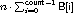entries: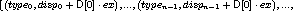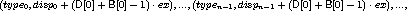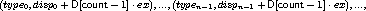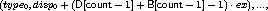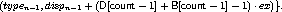A call to MPI_TYPE_VECTOR(count, blocklength, stride, oldtype, newtype) is equivalent to a call to MPI_TYPE_INDEXED(count, B, D, oldtype, newtype) where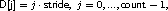and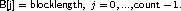Hindexed The function MPI_TYPE_CREATE_HINDEXED is identical to MPI_TYPE_INDEXED, except that block displacements in array_of_displacements are specified in bytes, rather than in multiples of the oldtype extent.
 MPI_TYPE_CREATE_HINDEXED( count, array_of_blocklengths, array_of_displacements, oldtype, newtype) IN count number of blocks --- also number of entries in array_of_displacements and array_of_blocklengths (nonnegative integer) IN array_of_blocklengths number of elements in each block (array of nonnegative integers) IN array_of_displacements byte displacement of each block (array of integer) IN oldtype old datatype (handle) OUT newtype new datatype (handle)

int MPI_Type_create_hindexed(int count, int array_of_blocklengths[], MPI_Aint array_of_displacements[], MPI_Datatype oldtype, MPI_Datatype *newtype)

MPI_TYPE_CREATE_HINDEXED(COUNT, ARRAY_OF_BLOCKLENGTHS, ARRAY_OF_DISPLACEMENTS, OLDTYPE, NEWTYPE, IERROR)
INTEGER COUNT, ARRAY_OF_BLOCKLENGTHS(*), OLDTYPE, NEWTYPE, IERROR

MPI::Datatype MPI::Datatype::Create_hindexed(int count, const int array_of_blocklengths[], const MPI::Aint array_of_displacements[]) const
This function replaces MPI_TYPE_HINDEXED, whose use is deprecated. See also Chapter Deprecated Functions . Assume that oldtype has type map, { (type0,disp0), ..., (typen-1, dispn-1) } , with extent ex. Let B be the array_of_blocklength argument and D be the array_of_displacements argument. The newly created datatype has a type map withentries: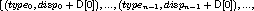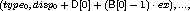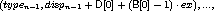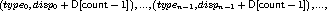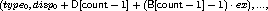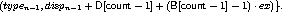Indexed_block

This function is the same as MPI_TYPE_INDEXED except that the blocklength is the same for all blocks. There are many codes using indirect addressing arising from unstructured grids where the blocksize is always 1 (gather/scatter). The following convenience function allows for constant blocksize and arbitrary displacements.

 MPI_TYPE_CREATE_INDEXED_BLOCK(count, blocklength, array_of_displacements, oldtype, newtype) IN count length of array of displacements (non-negative integer) IN blocklength size of block (non-negative integer) IN array_of_displacements array of displacements (array of integer) IN oldtype old datatype (handle) OUT newtype new datatype (handle)

int MPI_Type_create_indexed_block(int count, int blocklength, int array_of_displacements[], MPI_Datatype oldtype, MPI_Datatype *newtype)

MPI_TYPE_CREATE_INDEXED_BLOCK(COUNT, BLOCKLENGTH, ARRAY_OF_DISPLACEMENTS, OLDTYPE, NEWTYPE, IERROR)
INTEGER COUNT, BLOCKLENGTH, ARRAY_OF_DISPLACEMENTS(*), OLDTYPE, NEWTYPE, IERROR

MPI::Datatype MPI::Datatype::Create_indexed_block(int count, int blocklength, const int array_of_displacements[]) const

Struct MPI_TYPE_STRUCT is the most general type constructor. It further generalizes MPI_TYPE_CREATE_HINDEXED in that it allows each block to consist of replications of different datatypes.
 MPI_TYPE_CREATE_STRUCT(count, array_of_blocklengths, array_of_displacements, array_of_types, newtype) IN count number of blocks (nonnegative integer) --- also number of entries in arrays array_of_types, array_of_displacements and array_of_blocklengths IN array_of_blocklength number of elements in each block (array of nonnegative integer) IN array_of_displacements byte displacement of each block (array of integer) IN array_of_types type of elements in each block (array of handles to datatype objects) OUT newtype new datatype (handle)

int MPI_Type_create_struct(int count, int array_of_blocklengths[], MPI_Aint array_of_displacements[], MPI_Datatype array_of_types[], MPI_Datatype *newtype)

MPI_TYPE_CREATE_STRUCT(COUNT, ARRAY_OF_BLOCKLENGTHS, ARRAY_OF_DISPLACEMENTS, ARRAY_OF_TYPES, NEWTYPE, IERROR)
INTEGER COUNT, ARRAY_OF_BLOCKLENGTHS(*), ARRAY_OF_TYPES(*), NEWTYPE, IERROR

static MPI::Datatype MPI::Datatype::Create_struct(int count, const int array_of_blocklengths[], const MPI::Aint array_of_displacements[], const MPI::Datatype array_of_types[])
This function replaces MPI_TYPE_STRUCT, whose use is deprecated. See also Chapter Deprecated Functions .
Example Let type1 have type map,

{ ( double, 0), ( char, 8) } ,

with extent 16. Let B = (2, 1, 3), D = (0, 16, 26), and T = (MPI_FLOAT, type1, MPI_CHAR). Then a call to MPI_TYPE_STRUCT(3, B, D, T, newtype) returns a datatype with type map, { ( float, 0), ( float, 4), ( double, 16), ( char, 24), ( char, 26), ( char, 27), ( char, 28) } . That is, two copies of MPI_FLOAT starting at 0, followed by one copy of type1 starting at 16, followed by three copies of MPI_CHAR, starting at 26. (We assume that a float occupies four bytes.)

In general, let T be the array_of_types argument, where T[i] is a handle to, typemapi = { (type0i , disp0i ) , ... , (typeni-1i , dispni-1i ) } , with extent exi. Let B be the array_of_blocklength argument and D be the array_of_displacements argument.Let c be the count argument. Then the newly created datatype has a type map with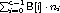entries: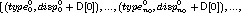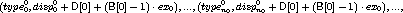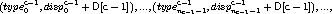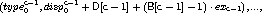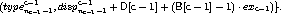A call to MPI_TYPE_CREATE_HINDEXED(count, B, D, oldtype, newtype) is equivalent to a call to MPI_TYPE_CREATE_STRUCT(count, B, D, T, newtype), where each entry of T is equal to oldtype.Up: Derived Datatypes Next: Subarray Datatype Constructor Previous: Type Constructors with Explicit Addresses